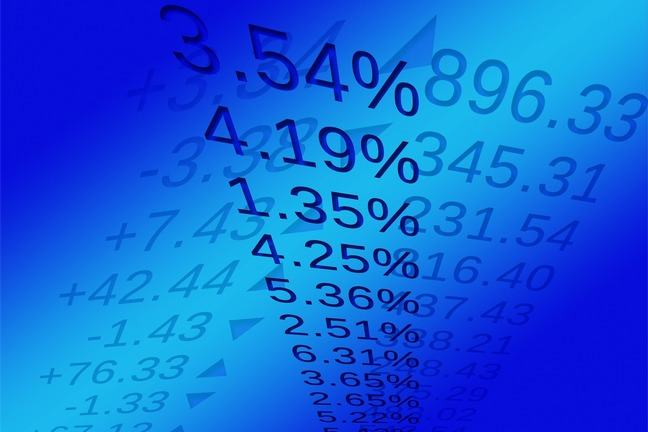## Want to keep learning?

This content is taken from the SOAS University of London's online course, Risk Management in the Global Economy. Join the course to learn more.
2.9

## SOAS University of London# The risk of financial assets

In the previous step you have seen how you can compute the expected return of a financial asset, and how you can measure the variability of its returns. Here, we look at one particular question, which is of great importance: what would happen to the riskiness of an asset, and to our measure of risk, if extreme returns became more likely?

Suppose there is a greater probability of observing large positive returns and large negative returns. Think about how the standard deviation of returns is calculated: the deviation of each return from the mean return is squared, and then multiplied by the probability of the return occurring. What will be the effect on the standard deviation if we increase the chances of extreme events?

We can examine this question by computing the expected return and standard deviation in an example. We believe the returns on asset $$A$$ follow this probability distribution:

Probability Return: $$R_A$$
0.10 –3%
0.30 2%
0.50 8%
0.10 12%

The extreme returns –3% and 12% have a probability of 0.10 = 10% each; there is a probability of 30% that the return on the security is 2%; and a probability of 50% that the return is 8%. Let’s first compute the expected return and standard deviation for this probability distribution. And then we will see what happens if we increase the probabilities of observing the extreme returns.

Remember we calculate the expected return by multiplying each possible return by the probability of the return occurring, and then adding these products together:

$\mu_A = (0.10 \times (-0.03)) + (0.30 \times 0.02) + (0.50 \times 0.08) + (0.10 \times 0.12) = 0.055 = 5.5\%$

The expected return is $$\mu_A = E(R_A) = 5.5\%$$.

### What is the standard deviation of the returns on asset $$A$$?

We first see how much each possible return deviates from the expected return, and then square those differences, as shown in the following table:

Probability $$R_A$$ - $$\mu_A$$ $$(R_A - \mu_A)^2$$
0.10 –0.03 – 0.055 = –0.085 0.007225
0.30 0.02 –0.055 = –0.035 0.001225
0.50 0.08 – 0.055 = 0.025 0.000625
0.10 0.12 – 0.055 = 0.065 0.004225

We sum these squared deviations (with each squared deviation multiplied by the probability of the return occurring) to get the variance:

$\sigma_A^2 = (0.10 \times 0.007225) + (0.30 \times 0.001225) + (0.50 \times 0.000625) + (0.10 \times 0.004225) = 0.001825$

The variance is measured in terms of the returns squared. Therefore we take the square root of the variance to get the standard deviation, which is measured in terms of the returns themselves:

$\sigma_A = \sqrt {{\sigma}^2_A} = \sqrt 0.001825 = 0.04272 = 4.272\%$

We can see that asset $$A$$ has an expected return of 5.5% and a standard deviation of 4.272%.

Now we can ask what would happen if the returns on the asset were to become “riskier”, with the extreme returns –3% and 12% becoming more likely?

Suppose the distribution of returns on the asset is now described by the following table:

Probability Return: $$R_A$$
0.20 –3%
0.20 2%
0.40 8%
0.20 12%

Can you see the lowest and highest returns are now more likely, each with a probability of 20% instead of 10%, and the middle returns are correspondingly less likely? Incidentally, have you noticed how the probabilities in the tables always add up to one? And the sum of the probabilities should not add up to more than one. Why is that?

The expected return $$\mu_A$$ is now:

$\mu_A = (0.20 \times (-0.03)) + (0.20 \times 0.02) + (0.40 \times 0.08) + (0.20 \times 0.12) = 0.054 = 5.4\%$

The expected return is similar, but slightly smaller than it was before.

To compute the variance we look again at the difference between each return and the expected return, and square that difference:

Probability $$R_A - \mu_A$$ $$(R_A - \mu_A)^2$$
0.20 –0.03 – 0.054 = –0.084 0.007056
0.20 0.02 – 0.054 = –0.034 0.001156
0.40 0.08 – 0.054 = 0.026 0.000676
0.20 0.12 – 0.054 = 0.066 0.004356

The variance is:

$\sigma_A^2 = 0.20 \times 0.007056 + 0.20 \times 0.001156 + 0.40 \times 0.000676 + 0.20 \times 0.004356 = 0.002784$

and the standard deviation is:

$\sigma_A = \sqrt {{\sigma}^2_A} = \sqrt 0.002784 = 0.05276 = 5.276\%$

In this second probability distribution we have increased the probability of observing the two extreme returns. The expected return of 5.4% is close to the expected return in the first case (5.5%). But the standard deviation of 5.276% is now higher (than 4.272%). The asset has become riskier, and our measure of risk reflects this.

Is that what you predicted?

Share your understanding and interpretation of risk and standard deviation by discussing this question in the comments section.